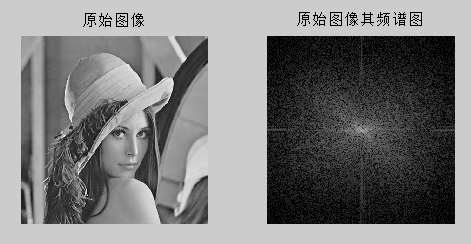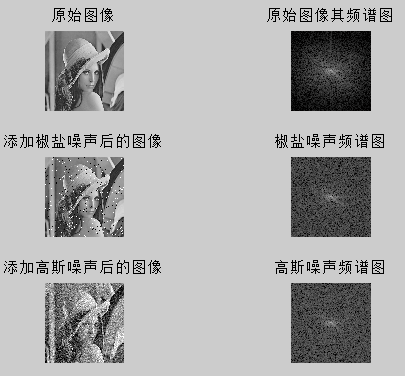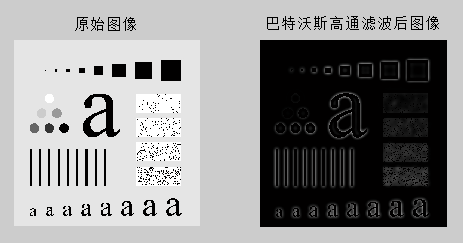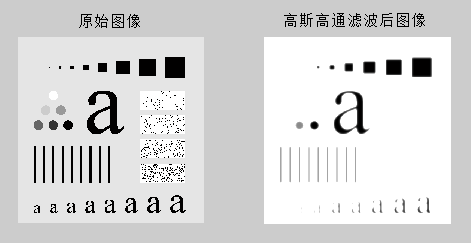• ## matlab数字图像的傅立叶变换实验

万次阅读 多人点赞 2016-11-11 10:57:53
实验三 图像的傅立叶变换 1.启动MATLAB程序，读入一幅图像；对图像做FFT。使用’subplot’命令，同时显示原始图像其频谱图； 1.1实验过程： 首先读取一幅图像，然后将这幅图像归一化到0~1之间，然后对图像做二维...
实验三  图像的傅立叶变换
1.启动MATLAB程序，读入一幅图像；对图像做FFT。使用’subplot’命令，同时显示原始图像其频谱图；
1.1实验过程：
首先读取一幅图像，然后将这幅图像归一化到0~1之间，然后对图像做二维离散傅立叶变换，然后做快速傅里叶变换，即直流分量移到频谱中心，让正半轴部分和负半轴部分的图像分别关于各自的中心对称，然后取傅立叶变换的实部，然后做频谱对数变换，就得到原图像的频谱图，最后将原图像和对应的频谱图显示出来。
1.2代码及图像
F=fft2(im2double(I)); %FFT
F=fftshift(F); %FFT频谱平移
F=real(F);
T=log(F+1); %频谱对数变换
subplot(1,2,1),imshow(I),title('原始图像');
subplot(1,2,2),imshow(T,[]),title('原始图像其频谱图');2. 读入一幅图像，分别为图像添加椒盐、高斯噪声，做FFT变换。使用’subplot’命令，将原始图像、原始图像频谱图、添加噪声后的图像，以及噪声图像的频谱图同时显示出来。
2.1实验过程
得到原始图像的频谱图的方法同上，然后对原图像模拟叠加密度为0.04的椒盐噪声，然后添加模拟均值为0方差为0.02的高斯噪声，然后得到噪声图像的频谱图，方法同上，最后显示所有图像。
2.2代码及图像
F=fft2(im2double(I)); %FFT
F=fftshift(F); %FFT频谱平移
F=real(F);
T=log(F+1); %频谱对数变换
subplot(3,2,1),imshow(I),title('原始图像');
subplot(3,2,2),imshow(T,[]),title('原始图像其频谱图');
S=imnoise(I,'salt & pepper', 0.04); %模拟叠加密度为0.04的椒盐噪声
K=fft2(im2double(S)); %FFT
K=fftshift(K); %FFT频谱平移
K=real(K);
T=log(K+1); %频谱对数变换
subplot(3,2,3),imshow(S),title('添加椒盐噪声后的图像');
subplot(3,2,4),imshow(T,[]),title('椒盐噪声频谱图');
G=imnoise(I,'gaussian',0,0.02);%模拟均值为0方差为0.02的高斯噪声，
H=fft2(im2double(G)); %FFT
H=fftshift(H); %FFT频谱平移
H=real(H);
T=log(H+1); %频谱对数变换
subplot(3,2,5),imshow(G),title('添加高斯噪声后的图像');
subplot(3,2,6),imshow(T,[]),title('高斯噪声频谱图');3. 读入一幅图像，对图像分别进行高斯低通、巴特沃兹低通、高斯高通和巴特沃兹高通频域滤波，比较其锐化和平滑效果；
3.1实验过程
对于高斯低通频域滤波，首先求原图像的频谱图，然后根据二维高斯低通滤波器(GLPF)定义，对频谱图做高斯低通滤波，使低频通过而使高频衰减，最后做快速傅里叶逆变换，结果发现滤波后的图像变模糊，比原始图像减少尖锐的细节部分而突出平滑过渡部分；对于巴特沃兹低通频域滤波，然后根据二级巴特沃思低通滤波器(BLPF)定义，对频谱图做巴特沃兹低通滤波，使低频通过而使高频衰减，最后做快速傅里叶逆变换，结果发现滤波后的图像变模糊，比原始图像减少尖锐的细节部分而突出平滑过渡部分；经对比图像后发现，经过巴特沃思低通滤波的图像比经过高斯低通频域滤波的图像更平滑。
对于高斯高通频域滤波，首先求原图像的频谱图，然后根据截频距原点为D0的高斯高通滤波器(GHPF)定义，对频谱图做高斯高通滤波，使高频通过而使低频衰减，最后做快速傅里叶逆变换，结果发现滤波后的图像变锐化，比原始图像减少平滑过渡而突出边缘等细节部分；对于巴特沃兹高通频域滤波，然后根据二阶且截至频率距原点的距离为D0的巴特沃思高通滤波器(BHPF)定义，对频谱图做巴特沃兹高通滤波，使高频通过而使低频衰减，最后做快速傅里叶逆变换，结果发现滤波后的图像变锐化，比原始图像减少平滑过渡而突出边缘等细节部分；经对比图像后发现，经过高斯低通滤波的图像比经过高斯低通巴特沃思低通频域滤波的图像更平滑。
3.2代码及图像
%高斯低通频域滤波
subplot(1,2,1),imshow(I),title('原始图像');
I=double(I);
S=fftshift(fft2(I));
[M,N]=size(S);
n=2;
d0=30; %GLPF滤波，d0=5，15，30（程序中以d0=30为例）
n1=floor(M/2);
n2=floor(N/2);
for i=1:M
for j=1:N
d=sqrt((i-n1)^2+(j-n2)^2);
h=1*exp(-1/2*(d^2/d0^2));
S(i,j)=h*S(i,j);
end
end
S=ifftshift(S);
S=uint8(real(ifft2(S)));
subplot(1,2,2),imshow(S),title('高斯低通滤波图像');
%巴特沃斯低通频域滤波
subplot(1,2,1),imshow(I),title('原始图像');
F=double(I);                % 数据类型转换，MATLAB不支持图像的无符号整型的计算
G=fft2(F);                    % 傅立叶变换
G=fftshift(G);                 % 转换数据矩阵
[M,N]=size(G);
nn=2;                       % 二阶巴特沃斯(Butterworth)低通滤波器
d0=30;                      %截止频率为30
m=fix(M/2); n=fix(N/2);
for i=1:M
for j=1:N
d=sqrt((i-m)^2+(j-n)^2);
h=1/(1+0.414*(d/d0)^(2*nn));         % 计算低通滤波器传递函数
result(i,j)=h*G(i,j);
end
end
result=ifftshift(result);
Y2=ifft2(result);
Y3=uint8(real(Y2));
subplot(1,2,2),imshow(Y3),title('巴特沃斯低通滤波')
%巴特沃斯高通频域滤波
subplot(121),imshow(I); title('原始图像');
F=double(I);% 数据类型转换，MATLAB不支持图像的无符号整型的计算
G=fft2(F);% 傅立叶变换
G=fftshift(G);%转换数据矩阵
[M,N]=size(G);
nn=2;% 二阶巴特沃斯(Butterworth)高通滤波器
d0=30;
m=fix(M/2);n=fix(N/2);
for  i=1:M
for j=1:N
d=sqrt((i-m)^2+(j-n)^2);
if (d==0)
h=0;
else
h=1/(1+0.414*(d0/d)^(2*nn));% 计算传递函数
end
result(i,j)=h*G(i,j);
end
end
result=ifftshift(result);
J2=ifft2(result);
J3=uint8(real(J2));
subplot(122),imshow(J3); title('巴特沃斯高通滤波后图像'); % 滤波后图像显示展开全文• ## 二维图像的傅立叶变换

万次阅读 多人点赞 2018-05-11 11:57:50
二维图像的傅立叶变换，与一维傅立叶相比，在理解上要抽象很多。我在网上找了几篇相对较好的文章，并用matlab自己做了几个实验图像，希望能对大家理解二维图像的傅立叶变换有所帮助。
摘要：二维图像的傅立叶变换，与一维傅立叶相比，在理解上要抽象很多。我在网上找了几篇相对较好的文章，并用matlab自己做了几个实验图像，希望能对大家理解二维图像的傅立叶变换有所帮助。
关键字：二维傅立叶变换，图像处理

本文是我旧博客中的博文，在CSDN图片显示不正常，请移步旧博客查看：https://imlogm.github.io/%E5%9B%BE%E5%83%8F%E5%A4%84%E7%90%86/image-fft/
1. 一维傅立叶变换
如果是理工科的话，一维傅立叶变换应该在大学里都学过。如果有所遗忘的话，可以看这篇比较易懂又不失数学性的文章"如何理解傅里叶变换公式？-马同学的回答"。
一维傅立叶变换的公式为：
$F(\omega)=\int_{-\infty}^{+\infty}f(t)e^{-i\omega t}dt$
2. 二维傅立叶变换
二维傅立叶变换的公式为：
$F(u,v)=\int_{-\infty}^{+\infty}\int_{-\infty}^{+\infty}f(x,y)e^{-i(ux+vy)}dxdy$
由一维傅立叶的公式，能比较容易类比得到二维傅立叶变换公式。但注意，二维傅立叶不是x方向与y方向正弦余弦的简单叠加，而是乘积的叠加。
比如：一维傅立叶变换的三角函数系是$sin(nx)$、$cos(nx)$以及常数1，二维傅立叶变换的三角函数系是$sin(ux+vy)$、$cos(ux+vy)$和常数1.而$sin(ux+vy)$和$cos(ux+vy)$可以继续分解为$sin(ux)sin(vy),\:\:sin(ux)cos(vy),\:\:cos(ux)sin(vy),\:\:cos(ux)cos(vy)$这四个乘积的形式。
如果对上面一段话的内容有兴趣的话，可以参看这篇文章"二维傅里叶变换是怎么进行的？-CharlyGordon的回答"。看不懂也没关系，只需要知道二维傅立叶不是x方向与y方向正弦余弦的简单叠加。

注意1：二维傅立叶变换后生成的图像与原图上的像素点不存在一一对应关系。原图中的像素值是x,y坐标轴下的（即空间域），而傅立叶变换后的像素值是u,v坐标轴下的（即频域）。

注意2：图像的像素点是离散且有限的，故实际进行图像傅立叶变换时，使用的是离散傅立叶变换（DFT），需要把上述公式中的积分号$\int_{-\infty}^{+\infty}$换成求和号$\sum$

3. 二维傅立叶变换的直观理解
原始图像经过二维傅立叶变换后得到的是u,v坐标系下的二维矩阵，由$(u_1,v_1)$、$(u_1,v_2)$、$(u_2,v_1)$等一系列点组成。每个位置$(u_n,v_n)$都有其对应的值$F(u_n,v_n)$。如果把这个二维矩阵归一化成傅立叶后的图像来显示，那么傅立叶后图像上像素点位置和像素点亮度就表征二维矩阵相应的点和该点的值。
如图1所示，有左中右三幅小图，我们先不管右小图，左小图是原始图像，中小图是傅立叶变换后的。可以看到傅立叶变换后的图片的两个斜对角出现了两个白点，这两点处的亮度值最大，其余点处亮度值为0。这表示原始图像可以由这两点所对应的三角波组成，三角波的幅值为其对应点的亮度。图1
那么这些三角波长什么样子呢？图2是我从网上找到的一幅图，原始出处未知。原来这张图是频移后的三角波，由于频移是下面才讲到的知识点，我感觉这样会对理解造成一定影响，所以我处理成频移前的，便于大家理解。相信对比图1和图2，你们能很快理解其中的关系。图2
在图2的右小图中，我用红框标出了其中的一个三角波，这个三角波的外形与图1的原始图像最相似。结果也和我们预料的一样，傅立叶变换后，这个三角波的幅值是最大的，所以我们看到了图1中小图左上角的白点。

注意：图2的三角波对应于图1中小图的左上角的区域，所以我们得到了左上角的白点。至于图1中小图的右上、左下、右下区域的三角波长什么样子，请看下面一段话。

看到这里可能大家还有个疑问：左上角的白点明白了，但是右下角的白点怎么来的？如果你自己动手做实验的话，会发现这两个白点是对称的（换而言之，右下角区域的三角波和左上角区域的三角波对称）。这其实是由两个原因共同作用造成的：其一，傅立叶双边频谱关于原点对称；其二，上面提到过，二维图像傅立叶变换是离散傅立叶变换，离散傅里叶变换本质是周期信号求傅里叶级数，所以其实会有周期延拓。
因为这两个性质涉及一些更深的知识，我不详细展开了。有兴趣的同学可以找信号处理方面的书来看，学过的同学应该能马上理解。
解决了图1中左小图、中小图的问题，那么图1的右小图是什么呢？右小图其实是中小图经过频移后的。为什么要频移，因为我们把傅立叶变换得到的二维矩阵用图像的方式显示时，默认的坐标原点(0, 0)位于图像的左上角。频移要做的就是把坐标原点移动到图像的中心。
可以想到，图1右小图的中间偏右下的点是由图1左小图左上角的点经过移动后得到的，而图1右小图的中间偏左上的点是由之前提到的“傅立叶双边频谱关于原点对称”这条性质得到的。
4. Matlab小实验
看了上面的内容，相信大家都已经对二维傅立叶有了一定的直观印象。我又用matlab写了个小程序，生成了几幅图片，帮助大家理解。图3图4图5图6图7图8
5. Matlab代码
最后附上我的matlab代码，便于大家自己做实验。
如果对傅立叶变换的代码有什么疑问的话，可以看这篇文章"使用matlab对图像进行傅里叶变换-三山音"。
% 用sin(x+y)的图像来帮助理解二维图像的傅立叶变换

img_size = 100;     % 图片尺寸
x_step = 1;
y_step = 1;
image = zeros(img_size, img_size);
for x = x_step:x_step:x_step*img_size
for y=y_step:y_step:y_step*img_size
image(x/x_step, y/y_step)=sin(4*pi*x/img_size + 4*pi*y/img_size);
end
end

subplot(1,3,1)
imshow(image)       % 原图
title('原图')

subplot(1,3,2)
image = im2double(image);
F_unshift = fft2(image);
F_unshift_abs = abs(F_unshift);
T = log(F_unshift_abs+1);
imshow(T);   % 傅立叶变换后，未频移前
title('傅立叶变换后，未频移')

subplot(1,3,3)
F = fftshift(F_unshift);
F_abs = abs(F);
T = log(F_abs+1);
imshow(T)           % 傅立叶变换后，频移后
title('傅立叶变换后，频移后')



展开全文• 使用matlab中自带fft等相关函数进行操作。代码如下：clear;clc;img=imread('test.jpg');img=rgb2gray(img);f=fft2(img); %傅里叶变换f=fftshift(f); %使图像对称r=real(f); %求图像频域实部i=imag(f); %求图像...
使用matlab中自带的fft等相关的函数进行操作。代码如下：clear;clc;img=imread('test.jpg');img=rgb2gray(img);f=fft2(img);        %傅里叶变换f=fftshift(f);      %使图像对称r=real(f);          %求图像频域实部i=imag(f);          %求图像频域虚部margin=log(abs(f));      %图像幅度谱，加log便于显示phase=log(angle(f)*180/pi);     %图像相位谱l=log(f);subplot(2,2,1),imshow(img),title('源图像');subplot(2,2,2),imshow(l,[]),title('频谱');subplot(2,2,3),imshow(margin,[]),title('幅度谱');subplot(2,2,4),imshow(phase,[]),title('相位谱');
展开全文• 数字图像处理是一门集计算机科学、光学、数学、物理学等多学科综合科学。随着计算机科学发展，数字图像处理技术取得了巨大进展，呈现出强大生命力，已经在多种领域...MATLAB作为数学领域应用最广泛一种软...
数字图像处理是一门集计算机科学、光学、数学、物理学等多学科的综合科学。随着计算机科学的发展，数字图像处理技术取得了巨大的进展，呈现出强大的生命力，已经在多种领域取得了大量的应用，推动了社会的发展。其中，遥感领域中，对于影像数据的处理均基于数字图像处理的技术。而遥感影像数据作为地理信息科学的重要数据源，如何从中获取有用的信息，是地理信息数据处理中重要的内容。MATLAB作为数学领域应用最广泛的一种软件，集成了对于图片处理的函数和功能，成为了处理数字图像问题的佼佼者。其出众的计算能力和简便的绘图能力可以有效进行数字图像的变换和操作。本文探究了MATLAB(R2014a)软件下数字图像处理部分简单内容方法的操作实现，包括图像变换、图像增强等。由于时间所迫和水平不足，对于本文中的问题请指出。在这里首先将介绍基本的操作。1　　数字图像处理基础1.1            MATLAB图像处理基本操作本文中对于大多数的操作，是对数字图像处理领域中最为著名的“lena”图片进行操作的。原图如下(Figure 1)：Figure 1首先，在MATLAB中显示这幅图片：123I=imread('lena.jpg');imfinfo('lena.jpg')imshow(I);其中，imread()可以读取图片，imfinfo()可以获取图片的信息(Figure 2)，imshow()可以显示图片。Figure 2从Figure 2中可以看出，该图片格式为png，长宽均为512px，颜色类型为真彩色。处理后的图片还需要进行保存：1imwrite(I,'lenaSave.jpg');1.2            图像数字化使用imread()读取图像后，可以看到，读入的图片I是以一个512*512*3的矩阵进行保存的，即分别是RGB颜色的数字图片。为了方便起见，在本文中，笔者将lena图进行了转换，转换为灰度图，即保存为一个512*512*1的矩阵。为了完成这样一个步骤，MATLAB中提供了相应的函数：12I1=rgb2gray(I);imshow(I1);I1以512*512*1的矩阵形式进行存储。效果如下图(Figure 3)：Figure 31.3            直方图为了显示图像灰度的分布情况，还需要绘制灰度直方图。可以使用如下代码：1figure,imhist(I1);效果如下(Figure 4)：Figure 41.4            图像噪声的添加为了完成多种图像处理的操作和试验，还可以对图片添加噪声。所用函数为imnoise (I, type)，该函数中的type可以为5种噪声参数，分别为：'gaussian'(高斯白噪声)，'localvar'(与图象灰度值有关的零均值高斯白噪声)，'poisson'(泊松噪声)，'salt & pepper'(椒盐噪声)和'speckle'(斑点噪声)。以下为高斯噪声(Figure 5)和椒盐噪声(Figure 6)的代码和效果：12I2=imnoise(I1,'gaussian');figure,imshow(I2);Figure 512I3=imnoise(I1,'salt & pepper');figure,imshow(I3);Figure 62         图像变换2.1            傅立叶变换傅立叶变换可以将图像从空间域转换到频率域，然后再进行相应的处理。MATLAB中有傅立叶变换的函数。原图：Figure 7傅立叶变换：123F=fft2(I1);S=abs(F);figure,imshow(S,[]);效果：Figure 8看上去是一片黑，事实上在全图的左上角可以看出有一个白点(即左上角缺失了)。平移：12Fc=fftshift(F);figure,imshow(abs(Fc),[]);效果：Figure 9将频率平移到中间。频谱图：1figure,imshow(uint8(abs(Fc/256)));效果：Figure 10之所以在本例中没有使用lena的图片，是因为该图傅立叶频谱图并无特点。傅立叶逆变换：123f=real(ifft2(F)/255);figure,imshow(f);效果：Figure 11经过逆变换后，仍然为原图。
展开全文• 1． 启动MATLAB程序，读入一幅图像；对图像做FFT。使用’subplot’命令，同时显示原始图像其频谱图； IenaImg=imread('lena.... %二维离散傅立叶变换 sfftI=fftshift(fftI); %直流分量移到频谱中心 RR=real(sfftI); %取
• 灰度和彩色图像的快速傅立叶变换：问题1：对输入的灰度和彩色图像进行快速傅立叶变换,显示频谱图; 问题2：进行逆变换,观察结果。 1、问题及说明 （1）对输入的灰度和彩色图像进行快速傅立叶变换,显示频谱图； ...
• 5通过本实验掌握利用MATLAB 编程实现数字图像的傅立叶变换。6、掌握怎样利用傅立叶变换进行频域滤波7、掌握频域滤波的概念及方法8、熟练掌握频域空间的各类滤波器9、利用MATLAB 程序进行频域滤波二、 实验原理1应用...
• 此次项目是在频谱域空间下操作，代码由c++（MFC）完成，没有使用matlab，完成 project04-01 到04-05 内容。包括快速傅立叶变换，频谱显示，低通滤波，高通滤波， 图像相关性匹配
• 第8章 图像傅立叶 变换 ;为什么要在频率域研究图像增强;为什么要在频率域研究图像增强;法国数学家傅立叶(生于1768年)在1822年出版的...1 一维连续函数的傅立叶变换FT;如果;离散函数f(x(其中xu=0,1,2,M-1)的傅 立叶变换;
• matlab 数字图像矩阵数据显示及其傅立叶变换
• 5. 通过本实验掌握利用MATLAB编程实现数字图像的傅立叶变换。二、 实验原理1.应用傅立叶变换进行图像处理傅里叶变换是线性系统分析的一个有力工具，它能够定量地分析诸如数字化系统、采样点、电子放大器、卷积滤波器...
• 注：本系列来自于图像处理课程实验，用Matlab实现最基本的图像处理算法 1.Fourier变换 （1）频域增强 除了在空间域内可以加工处理图像以外，我们还可以将...
• 主要介绍了傅立叶变换的算法，在matlab仿真环境下实现了图像的二维傅立叶变换
• 是基于matlab的数字图像的二维傅立叶变换(FFT)以及二维离散余弦DCT变换，提供里详细代码，.m文件，还包括讲解
• 本文简要介绍了数学上的傅立叶变换及其在AI中的应用。介绍傅里叶变换是有史以来最深刻的数学见解之一，但不幸的是，其含义深深地埋在了一些荒谬的方程式中。傅立叶变换是一种将某些东西分解为一堆正弦波的方法。 像...
• 傅立叶变换目标定位 and 卷积应用中目标特征识别 写这篇文章感觉就是一句话：我想验证：**时域中卷积操作等于频域中乘积，也就是这个公式：f*g(x) = F(x)*G(x)，**这句话。 直接上代码：每一行代码都有...
• ## 傅立叶变换－MATLAB

千次阅读 2016-03-29 21:39:22
2⃣️输入的图像是一个巨大的二维矩阵，利用matlab函数库中的fft2()命令对该矩阵进行二维离散傅里叶变换，得到图像的频谱。 3⃣️该频谱是一个复数矩阵，然后用取模函数abs()对该复数矩阵取模，得到振幅谱矩阵。 4...fft...

# 图像的傅立叶变换matlabmatlab 订阅